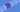# Python - Loops

### 2020-04-21

Let's get to some of the more exciting stuff in programs. One of the main reasons we write programs is to automate tasks. Let's say we have a list of salaries we want to count. This is a pretty simple example that can be solved in Excel, but can serve as a good introduction to loops in python. We will discuss two types of loops: While **and **For.

## While loops

``````salaires = [100, 200, 200]
totalSalaries  = 0

index = 0
while index < len(salaries):
totalSalaries += salareis[index]
index += 1``````

This first example show the use of a while loop. What we are saying here is "while the variable called index is less than the size of our salaries list, keep looping".

We are also accessing the list of salaired with the index. For example salaries[index] will start by being salaries which equals 100, the first item in our list.

A key thing to remember is to increment your index (or a different variable that you may use). For example, we have a line index += 1 to keep the index increasing. If we forgot this, our program would run forever (or at least until we stop the program :P).

Because of this issue, it is my recommendation that you try to use the **for **loop for most cases. Let's take a look at that.

## For Loops

``````salaires = [100, 200, 200]
totalSalaires = 0

for salary in salaires:
totalSalaries += salary ``````

We can see that this is a little more concise than the while loop. Also, we can use the in keyword to automcally grab the element in our list. So here we are saying, "for each salary in our salaries, do the following."

Python will give us each value of the list 100, 200, 200 in that order. We then add them each to our total.

Notice there is not neeed to worry about incrementing an index. So, this keep our programs safe and prevents us from getting into an infinite loop. While this is a benefit, sometimes you will need to use a **while **loop. Be sure to practice both :D.